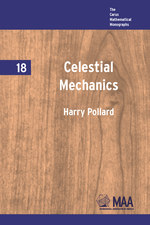# Celestial Mechanics

HARRY POLLARD
Volume: 18
Edition: 1
Pages: 145
https://www.jstor.org/stable/10.4169/j.ctt5hh9bd

1. Front Matter
(pp. i-vi)
2. PREFACE
(pp. vii-viii)
Harry Pollard
(pp. ix-x)
4. CHAPTER 1 THE CENTRAL FORCE PROBLEM
(pp. 1-56)

Celestial mechanics begins with the central force problem: to describe the motion of a particle of massmwhich is attracted to a fixed center0by a forcemf(r)which is proportional to the mass and depends only on the distancerbetween the particle and0.The functionfwill be called alaw of attraction.It is assumed to be continuous for$0 < r < \infty$

Mathematically, the problem is easy to formulate. Indicate the position of the mass by the vector r directed from0.According to Newton’s second law, the motion of the particle is governed by the...

5. CHAPTER 2 INTRODUCTION TO THE n-BODY PROBLEM
(pp. 57-92)

In then-body problem (better, then-particle problem) we are concerned with the motion ofnmass particles of masses${m_i}{\rm{ }}i = 1$, . . . ,nrespectively, attracting one another in pairs with the force$G{m_j}{m_k}r_{jk}^{ - 2}$where${r_{jk}}$is the distance between thekth andjth particle. We suppose that$n > 2.$

Let0represent an origin fixed in space and let${r_i},{v_i}$denote the position and velocity vectors of theith particle. Then, by Newton’s second law, thekth particle satisfies the equation

${m_k}{{\ddot r}_k} = \sum\limits_{j = 1 \hfill \atopj \ne k \hfill}^n {\frac{{G{m_j}{m_k}}}{{r_{jk}^2}}\frac{{{r_j} - {r_k}}}{{{r_{jk}}}}} where the right-hand side represents the total force exerted on the kth particle by the remaining... 6. CHAPTER 3 INTRODUCTION TO HAMILTON-JACOBI THEORY (pp. 93-132) We begin by recalling some basic facts from advanced calculus. Let the functions${y_k} = {y_k}\left( {{x_1}{\rm{, }}{\rm{. }}{\rm{. }}{\rm{. , }}{x_m}} \right),{\rm{ }}k = 1,{\rm{ }}{\rm{. }}{\rm{. }}{\rm{. , }}m$(1.1) denote a transformation of variables in anm-dimensional region. It will be supposed that each of the partial derivatives$\partial {y_k}/\partial {x_l}$exists and is continuous. The matrix m with entries$\partial {y_k}/\partial {x_l}$(k= row index,l= column index) is known as theJacobian matrixof the transformation; in more detail it is$\mathfrak = \left( {\begin{array}{*{20}{c}} {\frac{{\partial {y_1}}}{{\partial {x_1}}}} & {\frac{{\partial {y_1}}}{{\partial {x_2}}}} & \cdots & {\frac{{\partial {y_1}}}{{\partial {x_m}}}} \\ \vdots & {} & {} & {} \\ {\frac{{\partial {y_m}}}{{\partial {x_1}}}} & {\frac{{\partial {y_m}}}{{\partial {x_2}}}} & \cdots & {\frac{{\partial {y_m}}}{{\partial {x_m}}}} \\ \end{array}} \right)$The determinant of$\mathfrak{W}$, written$\left| \mathfrak{W} \right|$, is called the Jacobian of the transformation (1.1). It is known that if the transformation (1.1) carries a particular point$(x_1^0,{\text{ }}.{\text{ }}.{\text{ }}.{\text{ }},{\text{ }}x_m^0)$into the point$(y_1^0,{\text{ }}.{\text{ }}.{\text{ }}.{\text{ }},{\text{ }}y_m^0)\$and if the Jacobian does not vanish...

7. INDEX
(pp. 133-134)